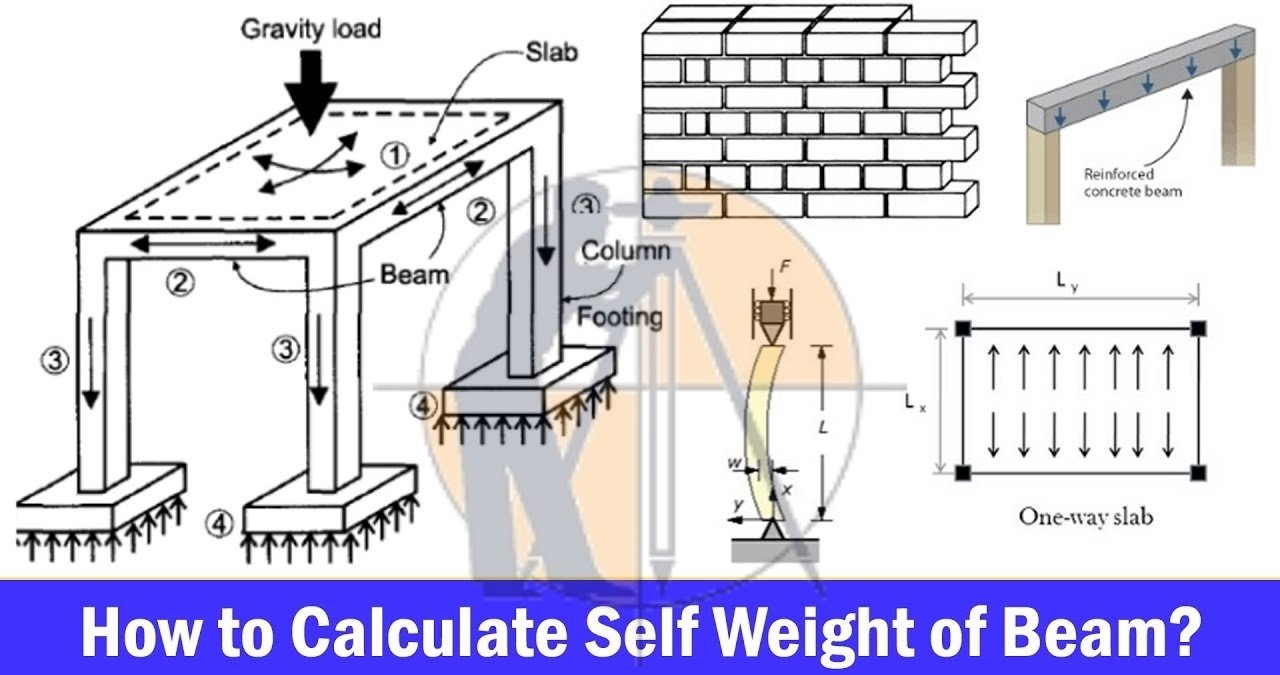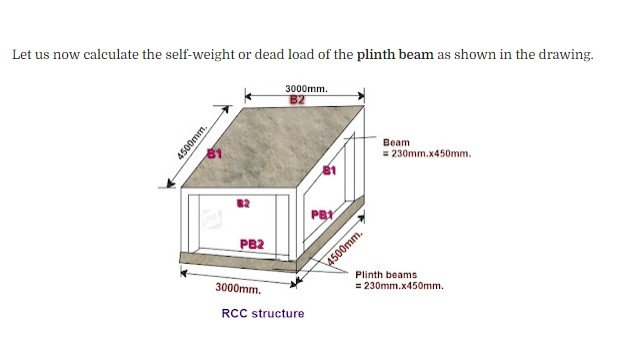Breaking News
Home / Civil Engineering / How to calculate the self weight of RCC footing And plinth beam

# How to calculate the self weight of RCC footing And plinth beam

## Calculate the self weight of R.C.C footings And Plinth Beam

In this Article we will discuss How To Calculate the Self weight or dead load of the R.C.C footing as shown in the drawing.## Given Data.

Length at the base = (L) = 750-mm.

Breadth at the base = (B) = 600-mm.

Total height (H) = 500-mm.

Top surface length = (l) = 450-mm.

Top surface width = (b) = 300-mm.

## Calculation.

The self weight of any R.C.C structure is calculated by the formula

[ Unit weight × ]

As you know, the unit weight of the R.C.C structure =  (D) = 2500-kg / cum.

The self-weight of R.C.C footing

= ( 2500-kg / m³ × 0.1904-m³ )

476-kg.

• ### Load Calculation On Column, Beam, Wall And Slab## Given Data.

The span of  the plinth beam-1 ( PB-1 ) = 4500-mm.=4.5-m.

The span of the plinth beam-2 ( PB-2 ) = 3000-mm.=3.0-m.

Sectional dimension of the plinth beams = 230-mm. × 450-mm.

= 0.23-m. × 0.45-m.

## Calculation.

The self weight of the (PB-2)

= [ Unit weight × (volume) ]

= [ 2500 kg / m³ × ( 3-m × 0.23-m. × 0.45-m) ]

776.25-kg.

The self weight of the (PB-1)

= [ Unit weight × (volume) ]

= [ 2500-kg/m³ × ( 4.5-m × 0.23-m. × 0.45-m) ]

= 1164.38-kg

## . Land Surveying & Architects

### THANKS.